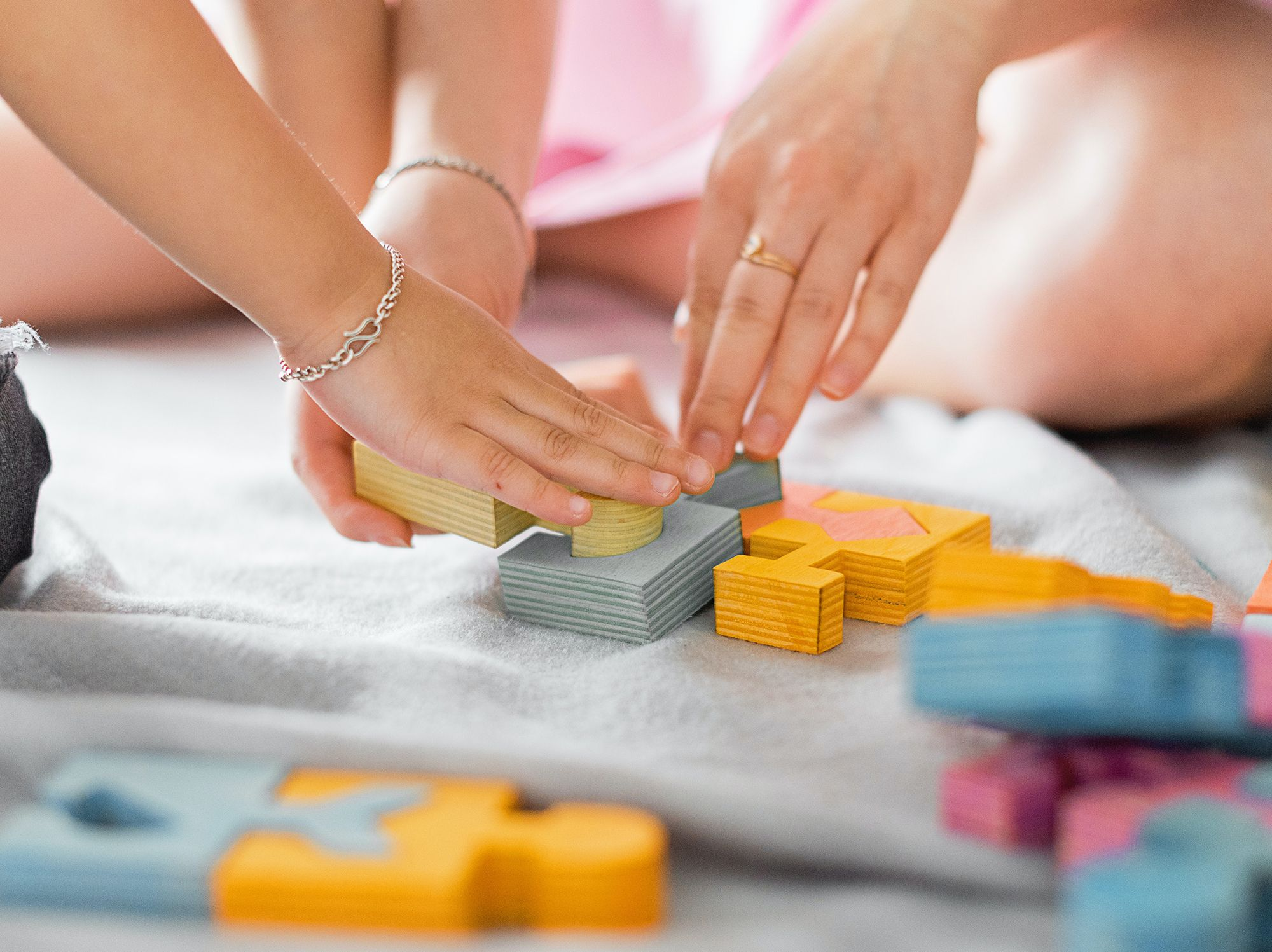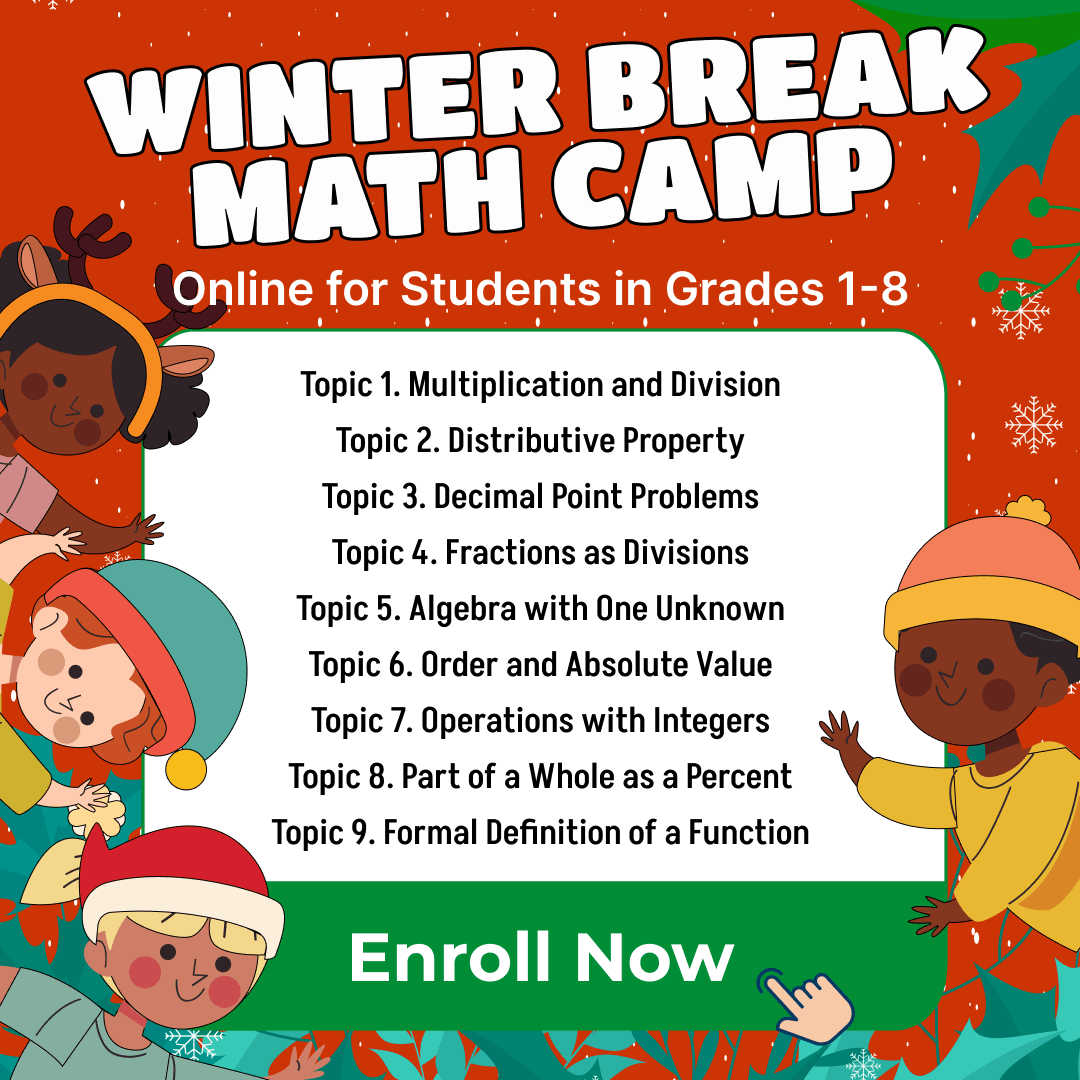# Check 7 Enjoyable Math Puzzles for Kids to Learn Why Your Child Needs Them

ReviewsPuzzles are excellent games for exercising one’s brains. Children need to develop their brainpower at the earliest stage, and puzzles can help achieve the best results. Puzzles drive learning and understanding of math, but they can quickly become boring. When children find puzzles dull, they zone out and stop learning, which is why you should update your arsenal of math games with fun math puzzles for kids mentioned in this article.

## Benefits of math puzzles for kids

Math is already challenging; so, you may wonder if you need to add the challenge of puzzles to the mix. However, math puzzles on their own are primarily beneficial to children, and these benefits explain why puzzles work well with math education. Here are four benefits of math puzzles.

### Math puzzles take away the fear of math

The complexity of math discipline can petrify even an average adult. To understand math, your child must first be fearless of studying it. Much of the adults’ fear of math can be traced to learning the subject in childhood. If you introduce math puzzles for kids at a child’s formative age, they will be less afraid of math because the twists and turns will become familiar.

Math for Kids

Is Your Child Struggling With Math?
1:1 Online Math Tutoring### Math puzzles improve strategic thinking

If you want to encourage critical thinking in your children, use math logic puzzles for kids. Such games help the mind to analyze problems and solve them, which is essential both in math and in everyday life. With every math puzzle your child completes, their understanding of math increases, and so does the kid’s ability to solve problems.

### Math puzzles encourage wholesome reasoning

Sometimes, when we teach children math, we offer them a one-dimensional approach to the problems. As we grow older, we realize that there is more than one way to solve a problem, which is something we should teach our child from their formative years. Interesting math puzzles for kids introduce a more wholesome approach to solving problems.

### Math puzzles improve the conversation around math

Kids don’t frequently discuss math among themselves, and their reasons are valid; math is definitely not an exciting subject. However, online math puzzles for kids can spark discussions about the discipline. Since such puzzles require a knowledge of math concepts, children will have to discuss math while brainstorming solutions.## Seven math puzzles for kids

As soon as you acknowledge the benefits of math puzzles for kids, the choice of the right math puzzle for your child should be your next step. There are a lot of options on the internet, so finding the right one may be a little challenging. Here are six of the best picks:

### Math Crossword Puzzles

Math crossword puzzles look like ordinary crossword puzzles, only this time, you would be matching numbers. Just like the clues under a crossword puzzle, you will have equations under a math crossword puzzle. These equations will be addition, subtraction, or multiplication problems that your kid will solve to progress through the dilemma. Printable math puzzles for kids like math crossword puzzles are easy to get on the internet.### KenKen

If you have ever come across Sudoku puzzles, KenKen will look familiar. The game has a grid-like style, and you are to use only the grid numbers to solve the problems. For example, if there are four boxes, your kid must use numbers 1, 2, 3, and 4 to play each row and column in that grid. For your child, this math puzzle may seem complicated at first, but it gets easier once they get the idea.

### Domino puzzle board

Domino math puzzle is a kids math puzzle that every child will enjoy. If you know how to play dominoes, you’ll love the Domino puzzle game as it follows the same rules. The only difference between a math domino and a regular domino is that with math dominoes, the kids won’t just pull tiles from the stack to match, they will be multiplying numbers or adding them to get the right tile.

### Finding the number pattern

Among the printable math puzzles kids love, you should try Finding the Number Pattern which requires your child to identify and replicate patterns. The game has numbers in random boxes on a 6×6 grid. The squares in the first row all have numbers in a specific arrangement, for example, in ascending order by 1. The subsequent rows will have some slots empty and require your child to fill the correct numbers based on the pattern they noticed in the first row. This puzzle is ideal for kids in the third grade and older.

Math for Kids

Is Your Child Struggling With Math?
1:1 Online Math Tutoring### 2048

2048 is one of the most straightforward online math puzzles for kids. You get a board with different numbers and multiply, add, subtract or divide them. The goal is to ensure that the only number left on the board after your calculations is 2048.

There is no wrong or right approach to this game. It is one of the best kids mathematics puzzles that you can teach your children to play. Your child can start dividing or adding numbers as they deem fit as long as they arrive at 2048 by the end.

### Magic square

The magic square is one of the printable math puzzles for kids. The square has a 3×3 grid, and your child has three different numbers in this grid. The sum of the numbers must be 15 either vertically, horizontally, or diagonally, and your child can only use a number once. Let your kid try any methods to reach the answer and encourage them every step of the way.

### Turn the fish

Unlike most options of math puzzles for kids online, you can play the Turn the Fish puzzle offline as long as you have the tools. In this puzzle, you will arrange matchsticks in a specific order to depict a fish facing a given direction. The kids have to turn only three matchsticks to get the fish to swim in the other direction.

They cannot overlap any sticks or move more than three of them. The kids have to keep trying till they figure out which sticks to move. Remember that this game has many strategies and correct solutions, so don’t box your students into a corner. Let them explore different approaches as long as they don’t give up.

## Conclusion

Math puzzles are fascinating and addictive, which isn’t necessarily bad, especially for a growing mind. The more time kids spend on children math puzzles, the more quickly they will understand and solve math problems. If you feel like joining in, you can solve these puzzles with your children. But if you’re too busy, then let the experienced tutors at Brighterly do the heavy lifting for you by using kids math puzzles in the learning process.

Winter Break Math Camp• Boost Math Skills this Winter Break at our Camp, Perfect for Students in Grades 1-8!

Winter Break Math Camp
Enhance Math Skills with Our Program, Perfect for 1st-8th Graders, Aligned with School Curriculum!